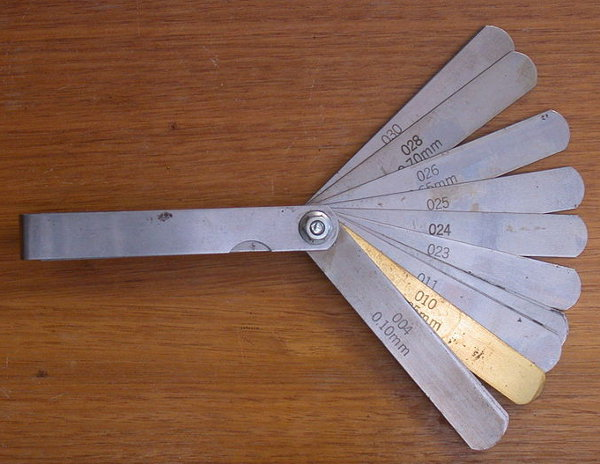Adding and subtracting decimals is the almost the same as adding and subtracting whole numbers.

Be careful...

• Line up the decimal point and the columns
• Fill in any blank decimal places with zeros.

What is the sum of 3.45 and 67.8? (Line up the columns. Fill in any blank decimal places with zeros.)

 3.45 + 67.80 71.25

## Example Two - Subtraction

What is the difference between 67.8 and 3.45? (Line up the columns. Fill in any blank decimal places with zeros.)

 67.80 – 3.45 64.35

## Question - Novelty Contact LensesAt c-r-a-z-y-e-y-e store, I bought 3 pairs of novelty contact lenses - Cats Eye for \$58.95, Rainbow Eye for \$38.78, and Green Dragon for \$29.55. How much change did I receive from \$150 cash?